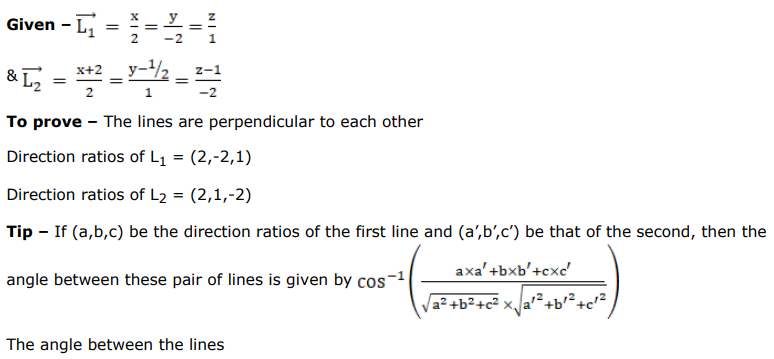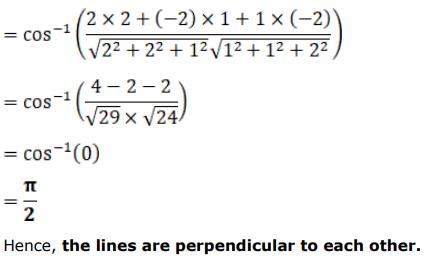# Solve this following

Question:

Show that the lines $x=-y=2 z$ and $x+2=2 y-1=-z+1$ are perpendicular to each other.

HINT: The given lines are $\frac{x}{2}=\frac{y}{-2}=\frac{z}{1}$ and $\frac{x+2}{1}=\frac{y-1 / 2}{1}=\frac{z-1}{-2}$.

Solution: